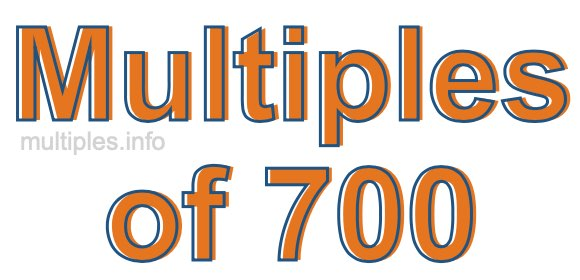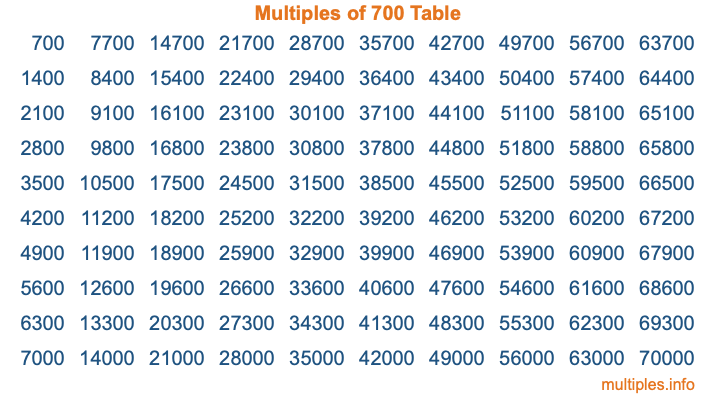Multiples of 700Welcome to the Multiples of 700 page. Here we will first teach you everything you will ever need to know about the multiples of 700, and then give you a study guide summary of everything we taught you to make sure you remember it all. Use this page to look up facts and learn information about the multiples of 700. This page will make you a multiples of seven hundred expert!

Definition of Multiples of 700
Multiples of 700 are all the numbers that when divided by 700 equal an integer. Each of the multiples of 700 are called a multiple. A multiple of 700 is created by multiplying 700 by an integer.

Therefore, to create a list of multiples of 700, you start with 1 multiplied by 700, then 2 multiplied by 700, then 3 multiplied by 700, and so on for as long as you want. Thus, the list of the first five multiples of 700 is 700, 1400, 2100, 2800, and 3500. To see a larger list of multiples of 700, see the printable image of Multiples of 700 further down on this page. We also have a category where you can choose any nth multiple of 700.

Multiples of 700 Checker
The Multiples of 700 Checker below checks to see if any number of your choice is a multiple of 700. In other words, it checks to see if there is any number (integer) that when multiplied by 700 will equal your number. To do that, we divide your number by 700. If the the quotient is an integer, then your number is a multiple of 700.

Is  a multiple of 700?

Least Common Multiple of 700 and ...
A Least Common Multiple (LCM) is the lowest multiple that two or more numbers have in common. This is also called the smallest common multiple or lowest common multiple and is useful to know when you are adding our subtracting fractions. Enter one or more numbers below (700 is already entered) to find the LCM.

Check out our LCM Calculator if you need more details about the Least Common Multiple or if you need the LCM for different numbers for adding and subtraction fractions.

nth Multiple of 700
As we stated above, 700 is the first multiple of 700, 1400 is the second multiple of 700, 2100 is the third multiple of 700, and so on. Enter a number below to find the nth multiple of 700.

th multiple of 700

Multiples of 700 vs Factors of 700
700 is a multiple of 700 and a factor of 700, but that is where the similarities end. All postive multiples of 700 are 700 or greater than 700. All positive factors of 700 are 700 or less than 700.

Below is the beginning list of multiples of 700 and the factors of 700 so you can compare:

Multiples of 700: 700, 1400, 2100, 2800, 3500, etc.

Factors of 700: 1, 2, 4, 5, 7, 10, 14, 20, 25, 28, 35, 50, 70, 100, 140, 175, 350, 700

As you can see, the multiples of 700 are all the numbers that you can divide by 700 to get a whole number. The factors of 700, on the other hand, are all the whole numbers that you can multiply by another whole number to get 700.

It's also interesting to note that if a number (x) is a factor of 700, then 700 will also be a multiple of that number (x).

Multiples of 700 vs Divisors of 700
The divisors of 700 are all the integers that 700 can be divided by evenly. Below is a list of the divisors of 700.

Divisors of 700: 1, 2, 4, 5, 7, 10, 14, 20, 25, 28, 35, 50, 70, 100, 140, 175, 350, 700

The interesting thing to note here is that if you take any multiple of 700 and divide it by a divisor of 700, you will see that the quotient is an integer.

Multiples of 700 Table
Below is an image of the first 100 multiples of 700 in a table. The table is in chronological order, column by column. The first column has the first ten multiples of 700, the second column has the next ten multiples of 700, and so on.The Multiples of 700 Table is also referred to as the 700 Times Table or Times Table of 700. You are welcome to print out our table for your studies.

Negative Multiples of 700
Although not often discussed or needed in math, it is worth mentioning that you can make a list of negative multiples of 700 by multiplying 700 by -1, then by -2, then by -3, and so on, to get the following list of negative multiples of 700:

-700, -1400, -2100, -2800, -3500, etc.

Multiples of 700 Summary
Below is a summary of important Multiples of 700 facts that we have discussed on this page. To retain the knowledge on this page, we recommend that you read through the summary and explain to yourself or a study partner why they hold true.

There are an infinite number of multiples of 700.

A multiple of 700 divided by 700 will equal a whole number.

700 divided by a factor of 700 equals a divisor of 700.

The nth multiple of 700 is n times 700.

The largest factor of 700 is equal to the first positive multiple of 700.

700 is a multiple of every factor of 700.

700 is a multiple of 700.

A multiple of 700 divided by a divisor of 700 equals an integer.

700 divided by a divisor of 700 equals a factor of 700.

Any integer times 700 will equal a multiple of 700.

Multiples of a Number
Here you can get the multiples of another number, all with the same attention to detail as we did for multiples of 700 on this page.

Multiples of
Multiples of 701
Did you find our page about multiples of seven hundred educational? Do you want more knowledge? Check out the multiples of the next number on our list!